# Growth indicatrix

(diff) ← Older revision | Latest revision (diff) | Newer revision → (diff)

indicator of an entire function

The quantity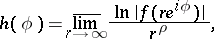characterizing the growth of an entire functionof finite order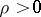and finite typealong the ray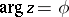for large(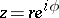). For instance, for the function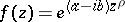the order isand the growth indicatrix is equal to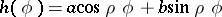; for the function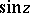the order is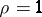and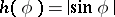. The functionis everywhere finite, continuous, has at each point left and right derivatives, has a derivative everywhere except possibly at a countable number of points,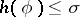always and there is at least onefor which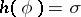, has the characteristic property of trigonometric convexity, i.e. if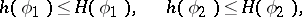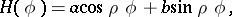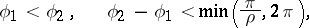then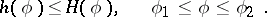The following inequality holds: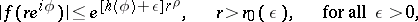where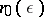is independent of.

The growth indicatrix is also introduced for functions that are analytic in an angle and in this angle are of finite order or have a proximate order and are of finite type.

How to Cite This Entry:
Growth indicatrix. Encyclopedia of Mathematics. URL: http://encyclopediaofmath.org/index.php?title=Growth_indicatrix&oldid=13310
This article was adapted from an original article by A.F. Leont'ev (originator), which appeared in Encyclopedia of Mathematics - ISBN 1402006098. See original article